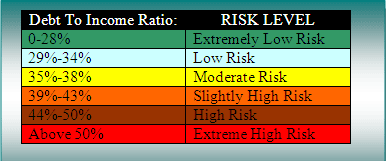# Risk Evaluation

Credit, Loan to Value and Debt to income are a few of the key factors in your qualification for a mortgage.  Read on to learn more about each key factor.

Debt To Income RatioDebt To Income Risk Analysis

The Debt to Income Ratio is calculated by dividing the total monthly payment by the total monthly income. Example:
If your family’s household income (for all borrowers) is \$10,000 per month gross before taxes are subtracted and your total monthly expenses including credit cards, auto loans, mortgage loans, home equity loans and any other installment or revolving debt total 3,000 per month then your Debt to income ratio would be 30%.

The Debt To Income (DTI) Ratio is derived by dividing total of monthly payments by gross monthly income. The lower your Debt To Income Ratio the lower your risk is to the lender.

Loan To Value

What is Loan To Value aka LTV? It’s the loan amount divided by the appraised value of your home. Loan to value demonstrates the percentage of the home that is leveraged. The Loan to Value is important for us to evaluate the risk the overall risk. The greater the Loan To Value percentage the greater the risk to the lender.

Loan To Value and Equity

Loan To Value (LTV) Ratio is derived by taking the new loan amount and dividing it by the homes appraised value. The lower the Loan To Value the lower the risk to the lender.

Equity is the determined by subtracting your homes value from the loans that have been borrowed against. The greater the equity the lower the risk. Example:
If your home appraises for \$100,000 and you want to borrow \$75,000 the loan to value ratio is 75%.

In the same scenario the equity would be determined by subtracting the loan amount of 75,000 from the appraised value of \$100,000 which would yield \$25,000 in equity.